﻿ 疫情期间的“消费与产出偏离之谜”：只是失业率问题吗？
>《财经研究》
2022第48卷第5期

1. 山东财经大学 财政税务学院, 山东 济南 250014;
2. 上海财经大学 高等研究院, 上海 200433

Mystery of the Deviation between Consumption and Output during the Period of COVID-19: Is It Just an Unemployment Issue?
Liu Jindong1, Ning Lei2, Jiang Lingzhen1
1. School of Public Finance & Taxation, Shandong University of Finance and Economics, Jinan 250014, China;
2. Institute for Advanced Research, Shanghai University of Finance and Economics, Shanghai 200433, China
Summary: During the period of COVID-19, consumption plummeted and deviated from output, showing the characteristics of speeding down and slow recovery. The abnormal phenomenon occurred not only in China, but also in other countries in the world. It is taken for granted that consumption growth declined more in the first quarter because of the limited population flow during the epidemic. However, with the cancellation of the home isolation policy and the resumption of work and production, the recovery of household consumption was still relatively slow when the unemployment rate did not rise significantly and family income was repaired rapidly. This overreaction of demand to the impact is difficult to be explained by the traditional consumption theory. According to the permanent income hypothesis, households have the motivation to smooth consumption, so the fluctuation of household consumption is less than that of income. In addition to the impact of the isolation policy, what other factors lead to the sharp decline of consumption, even more than the decline of output? What measures should the government take to deal with this situation? In this paper, we quantitatively explore the fundamental reason behind the puzzle thing that the consumption growth rate dropped more than the GDP growth rate during the epidemic in China using a heterogeneous agent life-cycle model. The model contains a variety of heterogeneities which include agent’s age, type of working firm, employment status, income status before unemployment, and the labor income risk. The main findings are as follows: First, the rising unemployment rate only accounts for 6.3% of the decline in consumption, while the declining employment quality under resource mismatch accounts for 26.1% and the increasing income uncertainty accounts for 29%. Second, both the rising unemployment rate and the increasing income uncertainty may recover in the short term, but the decline of employment quality caused by the mismatch of labor market resources will have a long-term impact. Third, the impacts on enterprises of different types and on individuals in different life cycles are heterogeneous. The income impact on middle-aged people in small and medium-sized enterprises is the most significant, while the consumption impact on young people in small and medium-sized enterprises is the most significant. The possible innovations of this paper lie in the following aspects: From the perspective of method, this paper constructs a heterogeneous agent life-cycle model, which considers the binary margin of income impact, the endogeneity of dynamic transfer of production efficiency and the heterogeneity of enterprises affected. Taking China’s economy before the epidemic as the initial steady state, this paper uses the method of counterfactual experiment to analyze the channels through which the epidemic affects household consumption, which is the first-time application in China. From the perspective of research expansion, this paper introduces the realistic research on the impact of unemployment rate on underemployment and thus on wage into the Chinese context. Combined with the empirical analysis with the micro data of China Urban Household Survey, it is verified that the rise of unemployment rate under the impact of economic crisis will aggravate the human resource mismatch, and resource mismatch will bring about greater wage penalty. As for policy inspiration, to boost consumption and restore the economy, countries around the world are running money printing machines to increase the stimulation of fiscal and monetary policies. This paper demonstrates that the slumped consumption is not only a short-term problem, but also a long-term lag effect. It is not only the problem of employment quantity, but also the problem of employment quality. Thus, on the premise of recognizing the internal causes of the sharp decline in consumption, this paper provides a directional reference for accurate and sustainable consumption promotion policies in the future.
Key words: heterogeneous agent life-cycle model    income uncertainty    underemployment    resource mismatch

2020年上半年，新冠疫情对我国经济发展造成一定冲击，一季度GDP增长率自改革开放以来首次出现负值，虽然二季度开始强势反弹，但整体经济活力仍处于缓慢恢复中。在这一过程中，一个可能反常的事实是消费变动远远超过了产出变动，其超速下降的同时还出现低速恢复的特征，2020年后三个季度GDP同比增长率分别恢复到3.2%、4.9%和6.5%，而社会消费品零售总额同比增长率则分别为−3.9%、0.9%和4.6%，在一系列刺激政策之下依然滞后于产出的恢复水平，最终的全年零售品销售总额仍然相比上一年是负增长。2021年上半年，在短期刺激政策下社会消费品零售表现出快速增长的态势，但在7月份开始又出现了总额回落，恢复速度低于预期。根据图1所示，以居民人均可支配收入和人均消费性支出来看，居民人均消费性支出也并没有随可支配收入同步变化，而是呈现出易跌难升的非对称特征。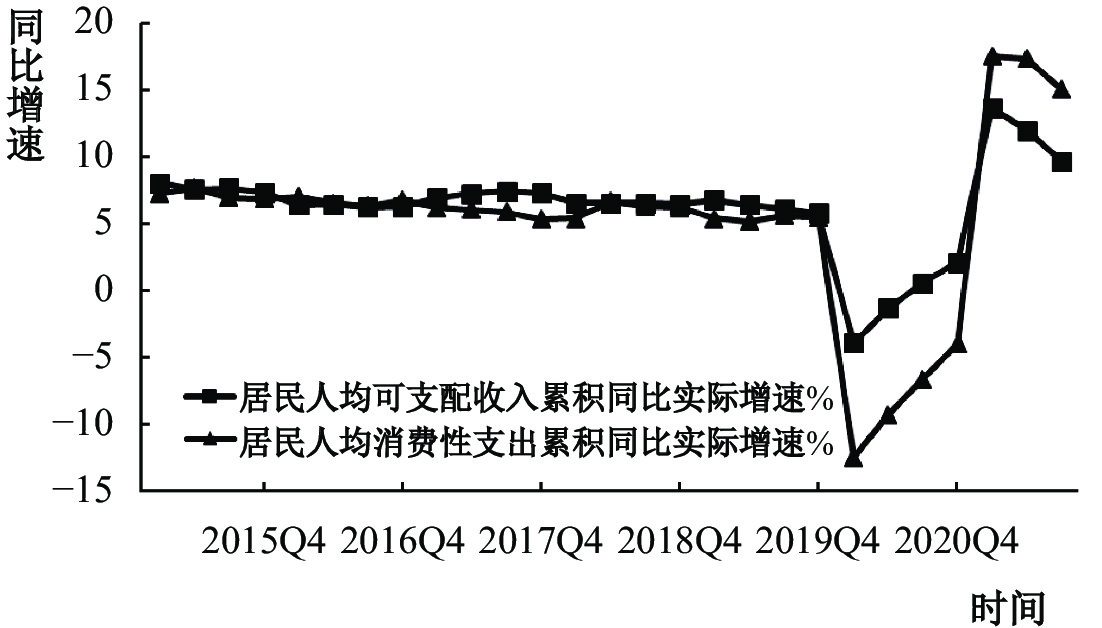图 1 中国人均可支配收入和消费性支出的变动趋势

（一）失业率对就业不足的影响分析

 被解释变量：是否就业不足 (1) (2) 失业率 1.491***(6.04) 3.912**(2.30) 个体特征 控制 控制 行业虚拟变量 控制 控制 省份虚拟变量 控制 控制 聚类标准误 控制 控制 样本数 48 646 1 487 注：（1）***、**分别表示1%、5%显著性水平下显著，下同；（2）括弧内为z统计值。

（二）就业不足的工资惩罚

 被解释变量：ln(工资收入) (1) (2) 是否就业不足 −0.166**(−2.19) −0.066 (−0.99) 失业率 −0.413 (−0.87) 0.088 (0.19) 个体特征 控制 控制 行业虚拟变量 控制 控制 省份虚拟变量 控制 控制 聚类标准误 控制 控制 样本数 1 501 1 714 注：括弧内为t统计值。

（一）人口结构与个体偏好

 $E\left[\sum _{j=1}^{J}{\beta }^{j}\left({\prod }_{i=1}^{j}{\phi }_{i}\right)U\left({c}_{j},{l}_{j}\right)\right]$ (1)

 $U\left({c}_{j},{l}_{j}\right)=\frac{{\left({c}^{\gamma }{l}^{1-\gamma }\right)}^{1-\sigma }}{1-\sigma }$ (2)

（二）个体的收入禀赋与最优化问题

 $V\left({a}_{j},{P}_{j},{S}_{j},{e}_{j}{,F}_{j}\right)=max\left[U\left({c}_{j},{l}_{j}\right)+\beta {\phi }_{j+1}EV\left({a}_{j+1},{P}_{j+1},{S}_{j+1},{e}_{j+1},{F}_{j}\right)\right]$ (3)

 ${\left(1+{\tau }_{c}\right)c}_{j}+{a}_{j+1}=\left(1+r\right){a}_{j}+{y}_{j}+Tr+beq$ (4)
 ${y}_{j}=\left\{\begin{array}{c}\left(1{-\tau }_{ss}{-\tau }_{ui}\right)w{\varepsilon }_{j}\left(1-{l}_{j}\right){P}_{j,S}\\ \left(1{-\tau }_{ss}{-\tau }_{ui}\right)w{\varepsilon }_{j}\left(1-{l}_{j}\right){P}_{j,F}\\ UI\end{array}\right.\begin{array}{c}if\;S=E\;and\;F=Small\\ if\;S=E\;and\;F=Big\\ if\;S=U\end{array}$ (5)

 $V\left({a}_{j}\right)=max\left[U\left({c}_{j},1\right)+\beta {\phi }_{j+1}EV\left({a}_{j+1}\right)\right]$ (6)

 ${\left(1+{\tau }_{c}\right)c}_{j}+{a}_{j+1}=\left(1+r\right){a}_{j}+{y}_{j}+Tr$ (7)
 ${y}_{j}=SS$ (8)

 $V\left({a}_{j},{P}_{j},{S}_{j},{e}_{j}{,F}_{j}\right)=max\left[U\left({c}_{j},{l}_{j}\right)+\beta {\phi }_{j+1}EV\left({a}_{j+1}\right)\right]$ (9)

 ${\left(1+{\tau }_{c}\right)c}_{j}+{a}_{j+1}=\left(1+r\right){a}_{j}+{y}_{j}+Tr$ (10)
 ${y}_{j}=\left\{\begin{array}{c}w{\varepsilon }_{j}\left(1-{l}_{j}\right){P}_{j,S}\\ w{\varepsilon }_{j}\left(1-{l}_{j}\right){P}_{j,F}\\ UI\end{array}\right.\begin{array}{c}if\;S=E\;and\;F=Small\\ if\;S=E\;and\;F=Big\\ if\;S=U\end{array}$ (11)

（三）政府行为

（四）企业行为

 ${Y}_{t}={A}_{t}{K}_{t}^{\alpha }{L}_{t}^{1-\alpha }$ (12)

 ${K}_{t+1}={\left(1-\delta \right)K}_{t}{+I}_{t}$ (13)

 ${r}_{t}=\alpha {A}_{t}{K}_{t}^{\alpha -1}{L}_{t}^{1-\alpha }-\delta$ (14)
 ${w}_{t}=\left(1-\alpha \right){A}_{t}{K}_{t}^{\alpha }{L}_{t}^{-\alpha }$ (15)

（一）人口参数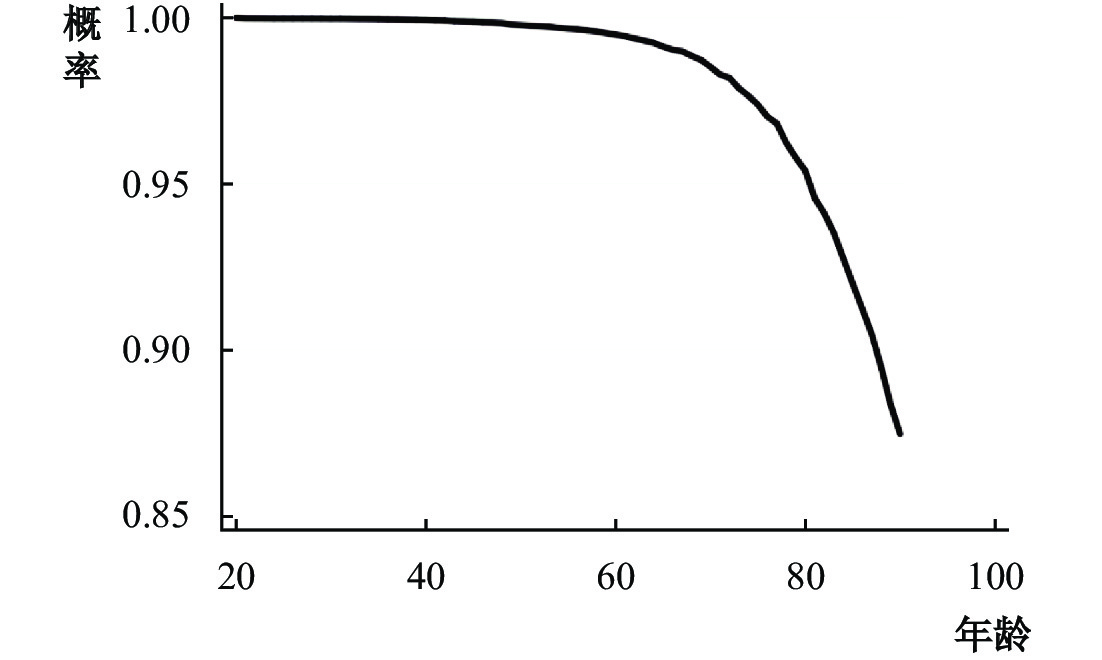图 2 条件生存概率

（二）收入禀赋

 $\log\left({Y}_{i}\right)={\alpha }_{0}+{\alpha }_{1}{age}_{i}+{\alpha }_{2}{age}_{i}^{2}+{\alpha }_{3}{X}_{i}+{\varepsilon }_{i}$ (16)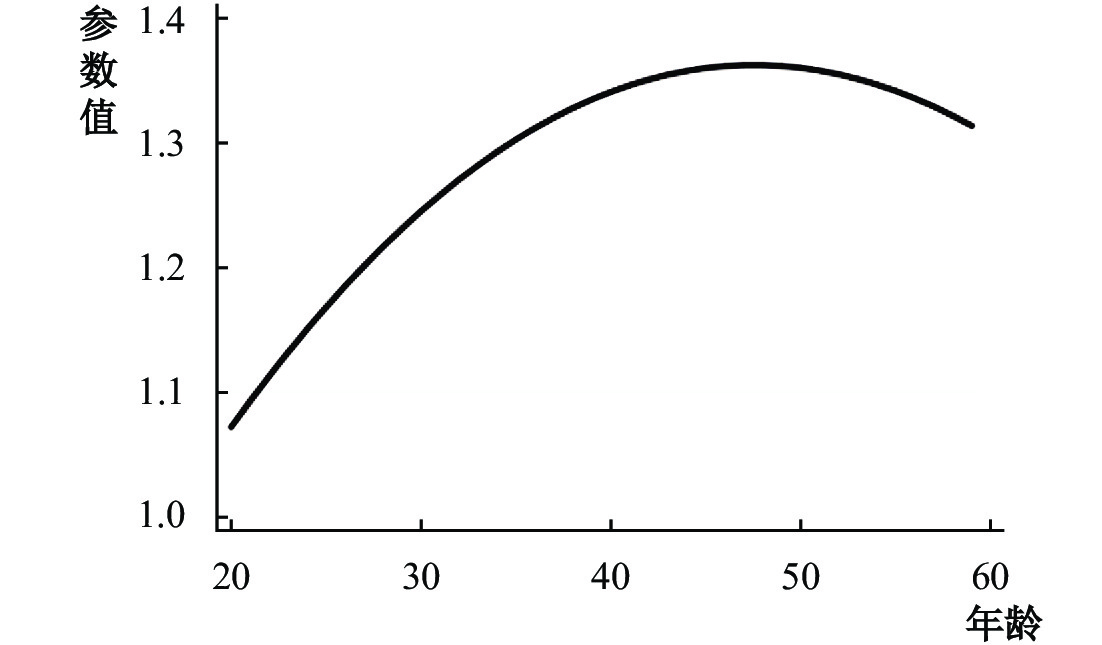图 3 年龄和效率参数

 ${P}_{it}=\rho {P}_{it-1}+{u}_{it}$ (17)

 $\left[\begin{array}{cc}\begin{array}{ccc}\pi & \left(1-\pi \right){q}_{1}& \left(1-\pi \right){q}_{2}\\ \pi & \left(1-\pi \right){p}_{\mathrm{1,1}}& \left(1-\pi \right){p}_{\mathrm{1,2}}\end{array}& \begin{array}{ccc}\left(1-\pi \right){q}_{3}& \cdots & \left(1-\pi \right){q}_{n}\\ \left(1-\pi \right){p}_{\mathrm{1,3}}& \cdots & \left(1-\pi \right){p}_{1,n}\end{array}\\ \begin{array}{ccc}\cdots & \cdots & \cdots \\ \pi & \left(1-\pi \right){p}_{n,1}& \left(1-\pi \right){p}_{n,2}\end{array}& \begin{array}{ccc}\cdots & \cdots & \cdots \\ \left(1-\pi \right){p}_{n,3}& \cdots & \left(1-\pi \right){p}_{n,n}\end{array}\end{array}\right]$ (18)

（三）政策及宏观变量参数

（四）效用函数参数

 具体内容 数学符号 数值 选取依据 贴现因子 $\beta$ 0.988 利率=4% 消费的权重 $\gamma$ 0.556 平均工作时间=0.45 相对风险规避系数 $\sigma$ 2.8 Frisch劳动供给弹性=0.5

 描述 具体内容 数学符号 数值 人口参数 人口增长率 $n$ 0.5% 条件生存概率 ${\phi }_{i}$ 2010人口普查 收入禀赋参数 大企业个体比例 $1-\mu$ 20% 中小企业个体比例 $\mu$ 80% 失业率 $\pi$ 4% 年龄和效率参数 ${\varepsilon }_{j}$ CFPS 2018 收入冲击 自相关系数 $\rho$ 0.8286 方差 ${\sigma }_{u}$ 0.05 政策参数 养老金替代率 $SS$ 0.45 失业保险替代率 $UI$ 0 商品消费税率 ${\tau }_{c}$ 11.5% 资本产出弹性 资本份额 $\alpha$ 0.5 折旧率 $\delta$ 0.1

（五）基准模型的适用性

（一）整体影响

 $\left[\begin{array}{cc}\begin{array}{ccc}\pi & \dfrac{\left(1-\pi \right){q}_{1}}{{q}_{1}+{q}_{2}+{q}_{3}}& \dfrac{\left(1-\pi \right){q}_{2}}{{q}_{1}+{q}_{2}+{q}_{3}}\\ \pi & \left(1-\pi \right){p}_{\mathrm{1,1}}& \left(1-\pi \right){p}_{\mathrm{1,2}}\end{array}& \begin{array}{ccc}\dfrac{\left(1-\pi \right){q}_{3}}{{q}_{1}+{q}_{2}+{q}_{3}}& 0& 0\\ \left(1-\pi \right){p}_{\mathrm{1,3}}& \cdots & \left(1-\pi \right){p}_{1,n}\end{array}\\ \begin{array}{ccc}\cdots & \cdots & \cdots \\ \pi & \left(1-\pi \right){p}_{n,1}& \left(1-\pi \right){p}_{n,2}\end{array}& \begin{array}{ccc}\cdots & \cdots & \cdots \\ \left(1-\pi \right){p}_{n,3}& \cdots & \left(1-\pi \right){p}_{n,n}\end{array}\end{array}\right]$ (19)

 大企业员工 中小企业员工 疫情前 疫情后 疫情前 疫情后 失业率 4% 4% 4% 6.54% 收入冲击 $\rho$ $\rho$ $\rho$ $\rho$ $\rho$ 收入冲击 ${\sigma }_{u}$ ${\sigma }_{u}$ ${\sigma }_{u}$ $1.7 \times {\sigma }_{u}$ ${1.7 \times \sigma }_{u}$ 转移矩阵 外生 内生 外生 内生

 变量描述 疫情前 疫情后 下降程度(%) 实际数据(%) 解释能力(%) 总产出 1.354 1.179 12.9 12.9 消费 0.847 0.701 17.3 24 72%

（二）生命周期性质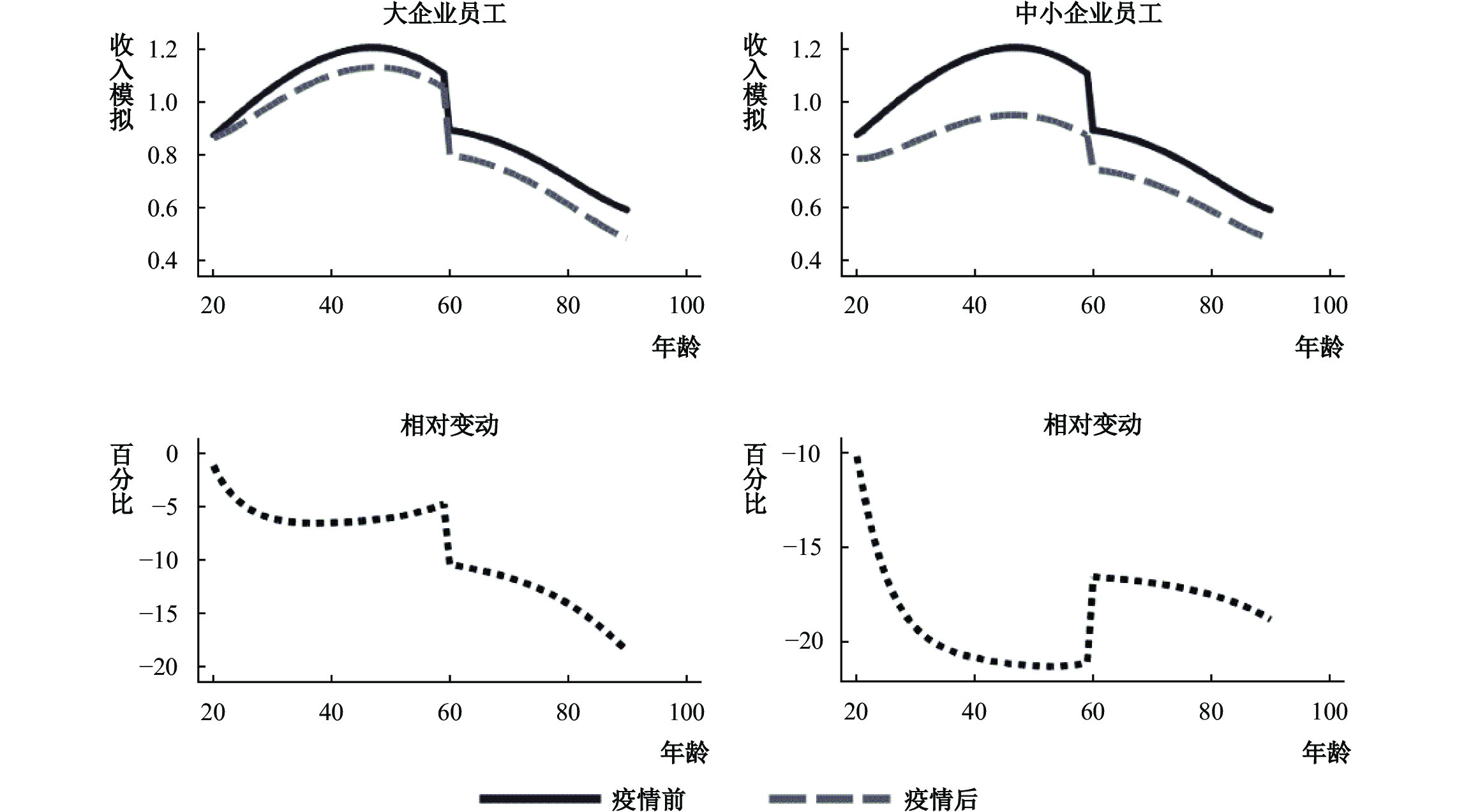图 4 疫情前后生命周期上个体收入的比较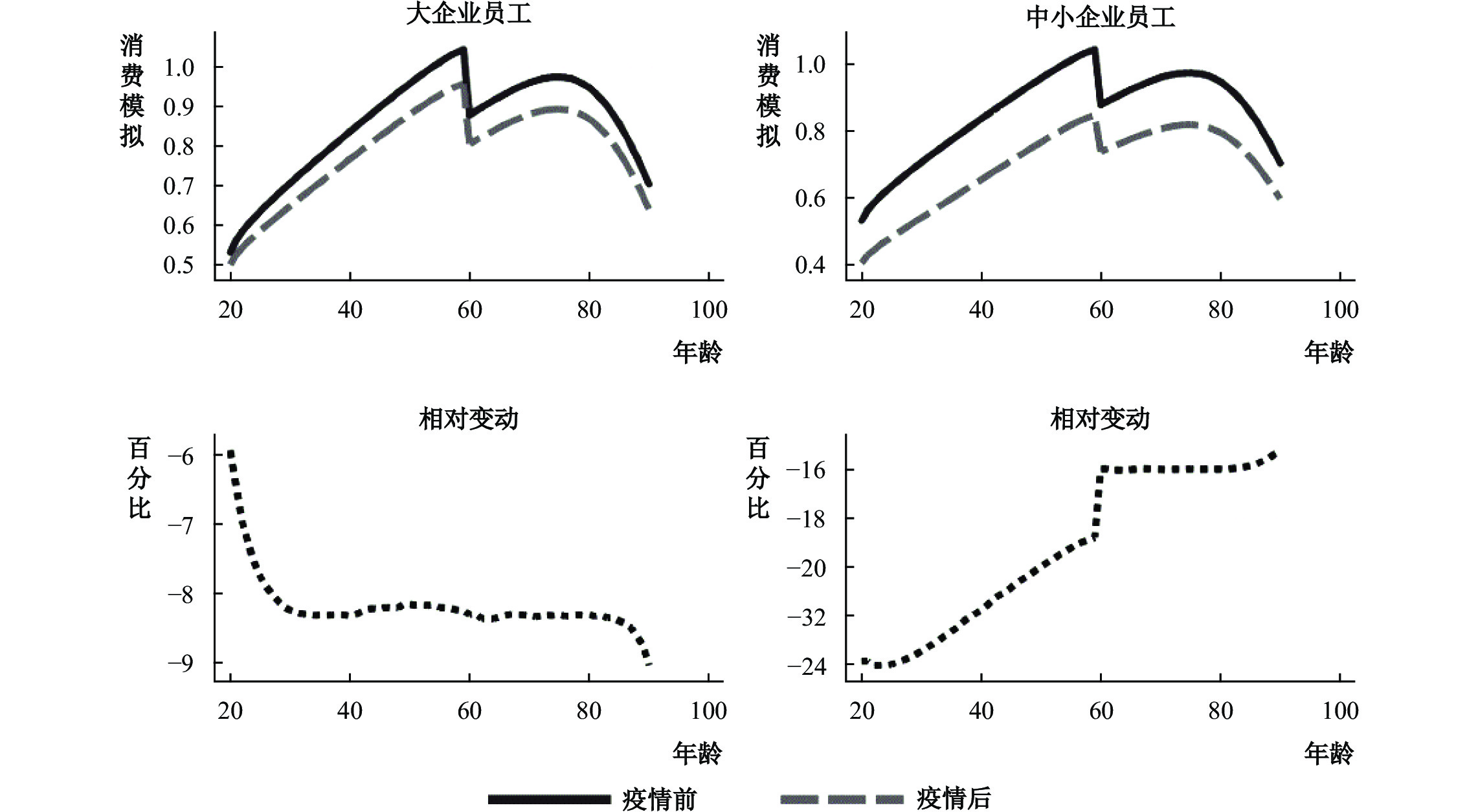图 5 疫情前后在生命周期上个体消费的比较

（三）不同因素的边际影响

 情景描述 下降程度：模型(%) 下降程度：实际数据(%) 解释能力(%) 总产出 消费 总产出 消费 总产出 消费 不确定性增加 3.8 7.0 12.9 24 29.4 29.0 广延边际失业率上升 1.1 1.5 12.9 24 8.7 6.3 集约边际配置效率降低 5.1 6.3 12.9 24 39.2 26.1 疫情后整体 12.9 17.3 12.9 24 100 72

（四）政策分析

2020年初的疫情对我国经济造成了严重冲击，其中一个反常的现象就是消费增速的下滑程度超过了产出增速的下滑程度。本文通过构建一个动态随机一般均衡模型，对这种现象进行了深入探讨，发现家庭收入不确定性增加和就业质量下降导致的劳动力市场资源错配是产生这一现象的重要原因，单纯的失业率上升仅会起到触发作用。其中，劳动力市场资源错配问题将会造成长期影响。同时，本文也发现疫情对于大企业和中小企业就业人员的影响具有很强的异质性。具体而言，由于大企业员工受到的失业风险和收入不确定性较小，因此疫情主要通过社会平均工资来影响退休后的收入。但对于中小企业员工而言，由于劳动力市场的疲软导致的收入结构性变化具有累积作用，这就导致中年群体受到疫情的影响最大。在当前需求收缩、供给冲击和预期转弱的压力下，本文的研究结论具有如下几点政策启示：

① 在本文的模型中，实际决策者以家庭为单位，因此本文中的“个体”和“家庭”是互用的。

② 因篇幅所限，竞争均衡的具体定义省略，感兴趣的读者可以向作者索取。

③ 我们也尝试过将收入冲击的格点选为9和19，发现结果基本没有变化。

④ 我们也尝试通过资本产出比校准 $\beta$ ，发现结果不影响我们的结论。

⑤ 具体年化规则为：假定一周有七天，每天有16小时可供支配。

⑥ 限于篇幅，分析图省略，读者若有兴趣可向作者索取。

⑦ 限于篇幅，分析表格省略，读者若有兴趣可向作者索取。

⑧ 参见Cooley和Prescott（1995）或者 Auerbach和Kotlikoff（1987）对如何选取相对风险规避系数的讨论。

  黄少卿, 陈彦. 中国僵尸企业的分布特征与分类处置[J]. 中国工业经济, 2017(3): 24–43.  李成友, 孙涛, 王硕. 人口结构红利、财政支出偏向与中国城乡收入差距[J]. 经济学动态, 2021(1): 105–124.  马虹, 李杰. 战略性的企业社会责任投资与市场竞争——基于Hotelling模型的分析框架[J]. 经济学动态, 2014(8): 78–89.  宁磊, 郑春荣. 延迟退休会提高社会福利水平吗?[J]. 财经研究, 2016(8): 27–39.  潘敏, 张新平. 新冠疫情、宏观经济稳定与财政政策选择——基于动态随机一般均衡模型的研究[J]. 财政研究, 2021(5): 38–54.  温兴祥. 失业、失业风险与农民工家庭消费[J]. 南开经济研究, 2015(6): 110–128.  肖兴志, 张伟广, 朝镛. 僵尸企业与就业增长: 保护还是排挤?[J]. 管理世界, 2019(8): 69–83. DOI:10.3969/j.issn.1002-5502.2019.08.006  赵达, 沈煌南, 张军. 失业率波动对就业者家庭消费和配偶劳动供给的冲击[J]. 中国工业经济, 2019(2): 99–116.  朱武祥, 张平, 李鹏飞, 等. 疫情冲击下中小微企业困境与政策效率提升——基于两次全国问卷调查的分析[J]. 社会科学文摘, 2020(6): 5–7.  Aguiar M, Gopinath G. Emerging market business cycles: The cycle is the trend[J]. Journal of Political Economy, 2007, 115(1): 69–102. DOI:10.1086/511283  Auerbach A J, Kotlikoff L J. Dynamic fiscal policy[M]. Cambridge: Cambridge University Press, 1987.  Bai C E, Hsieh C T, Qian Y Y. The return to capital in China[J]. Brookings Papers on Economic Activity, 2006, 37(2): 61–101.  Baker S R, Farrokhnia R A, Meyer S, et al. How does household spending respond to an epidemic? Consumption during the 2020 COVID-19 Pandemic[R]. NBER Working Paper No. 26949, 2020.  Barnichon R, Zylberberg Y. Underemployment and the trickle-down of unemployment[J]. American Economic Journal:Macroeconomics, 2019, 11(2): 40–78. DOI:10.1257/mac.20160220  Bourquin P, Delestre I, Joyce R, et al. The effects of coronavirus on household finances and financial distress[R]. IFS Briefing Note. BN298, 2020.  Carvalho V M, Garcia J R, Hansen S, et al. Tracking the COVID-19 crisis with high-resolution transaction data[R]. Cambridge Working Papers in Economics 2030, 2020.  Chetty R, Friedman J N, Hendren N, et al. How did COVID-19 and stabilization policies affect spending and employment? A new real-time economic tracker based on private sector data[R]. NBER Working Paper No. 27431, 2020.  Coibion O, Gorodnichenko Y, Weber M. The cost of the covid-19 crisis: Lockdowns, macroeconomic expectations, and consumer spending[R]. NBER Working Paper No. 27141, 2020.  Conesa J C, Krueger D. Social security reform with heterogeneous agents[J]. Review of Economic Dynamics, 1999, 2(4): 757–795. DOI:10.1006/redy.1998.0039  Cox N, Ganong P, Noel P, et al. Initial impacts of the pandemic on consumer behavior: Evidence from linked income, spending, and savings data[R]. University of Chicago, Becker Friedman Institute for Economics Working Paper No. 2020-82, 2020.  Deaton A, Paxson C. Intertemporal choice and inequality[J]. Journal of Political Economy, 1994, 102(3): 437–467. DOI:10.1086/261941  Fujita S. Declining labor turnover and turbulence[J]. Journal of Monetary Economics, 2018, 99: 1–19. DOI:10.1016/j.jmoneco.2017.12.005  He H, Ning L, Zhu D M. The impact of rapid aging and pension reform on savings and the labor supply[R]. International Monetary Fund Working Paper No. 19/61, 2019.  Hoke S H, Känzig D R, Surico P. Consumption in the time of COVID-19: Evidence from UK transaction data[R]. CEPR Discussion Paper No. 14733, 2020.  Imrohoroglu A, Imrohoroglu S, Joines D H. A life cycle analysis of social security[J]. Economic Theory, 1995, 6(1): 83–114. DOI:10.1007/BF01213942  Marinescu I, Skandalis D. Unemployment insurance and job search behavior[J]. The Quarterly Journal of Economics, 2021, 136(2): 887–931. DOI:10.1093/qje/qjaa037  Ortego-Marti V. Unemployment history and frictional wage dispersion[J]. Journal of Monetary Economics, 2016, 78: 5–22. DOI:10.1016/j.jmoneco.2015.12.002  Palmon O, Sun H L, Tang A P. Layoff announcements: Stock market impact and financial performance[J]. Financial Management, 1997, 26(3): 54–68. DOI:10.2307/3666213  Schmieder J F, Von Wachter T, Bender S. The effect of unemployment benefits and nonemployment durations on wages[J]. American Economic Review, 2016, 106(3): 739–777. DOI:10.1257/aer.20141566  Tauchen G. Finite state Markov-chain approximations to univariate and vector autoregressions[J]. Economics Letters, 1986, 20(2): 177–181. DOI:10.1016/0165-1765(86)90168-0